## Описание презентации International Trade : Theory and Policy Lecture 12 по слайдам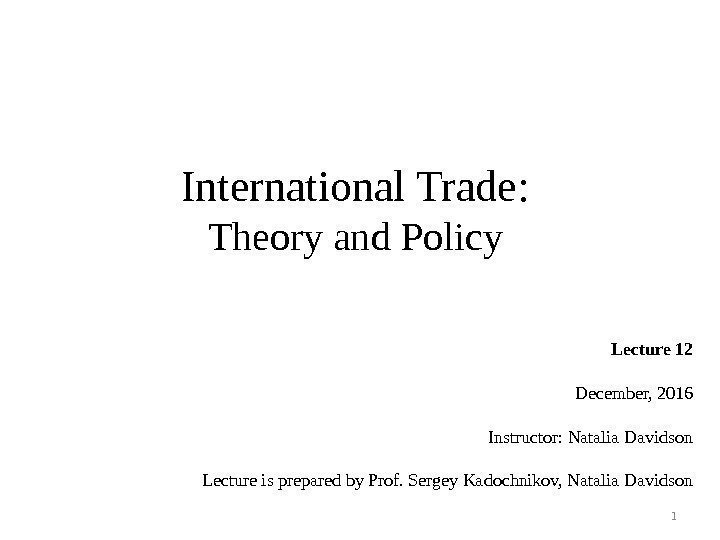International Trade : Theory and Policy Lecture 12 December, 2016 Instructor: Natalia Davidson Lecture is prepared by Prof. Sergey Kadochnikov, Natalia Davidson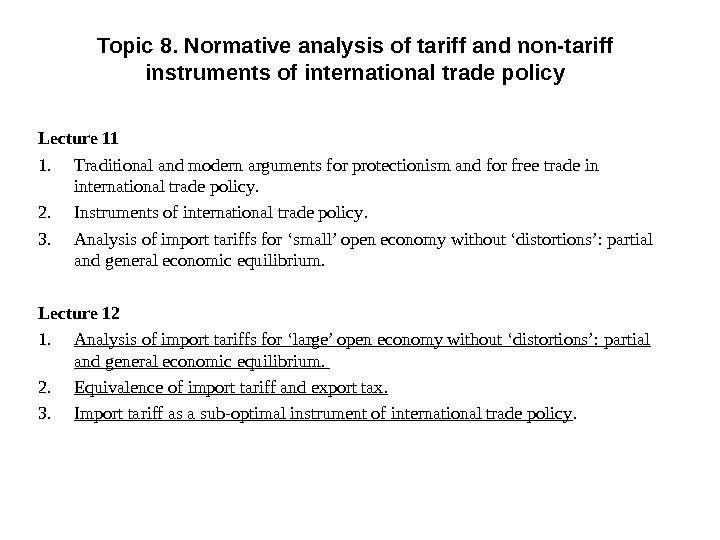Topic 8. Normative analysis of tariff and non-tariff instruments of international trade policy Lecture 11 1. Traditional and modern arguments for protectionism and for free trade in international trade policy. 2. Instruments of international trade policy. 3. Analysis of import tariffs for ‘small’ open economy without ‘distortions’: partial and general economic equilibrium. Lecture 12 1. Analysis of import tariffs for ‘large’ open economy without ‘distortions’: partial and general economic equilibrium. 2. Equivalence of import tariff and export tax. 3. Import tariff as a sub-optimal instrument of international trade policy.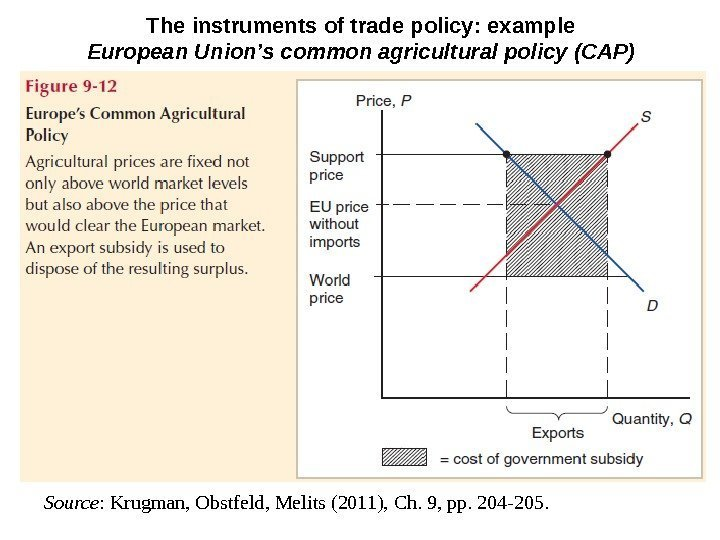The instruments of trade policy: example European Union’s common agricultural policy (CAP) Source : Krugman, Obstfeld, Melits (2011), Ch. 9, pp. 204 -205.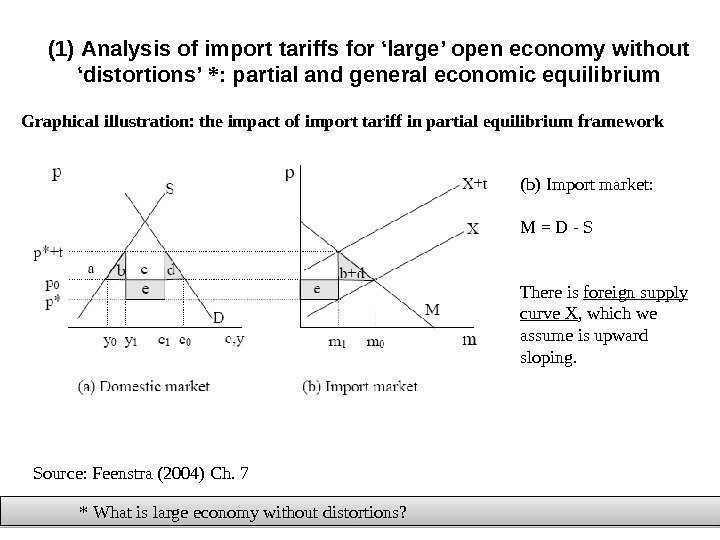( 1 ) Analysis of import tariffs for ‘large’ open economy without ‘distortions’ * : partial and general economic equilibrium Graphical illustration : the impact of import tariff in partial equilibrium framework * What is large economy without distortions ? Source: Feenstra (2004) Ch. 7 (b) Import market: M = D — S There is foreign supply curve X , which we assume is upward sloping.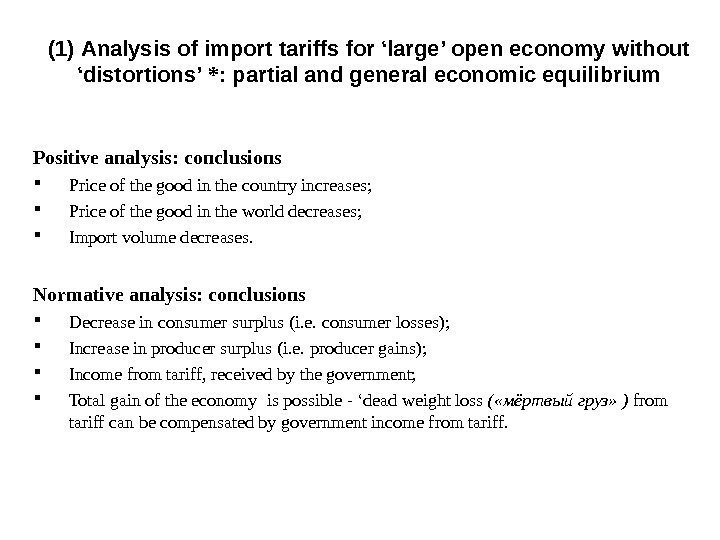( 1 ) Analysis of import tariffs for ‘large’ open economy without ‘distortions’ * : partial and general economic equilibrium Positive analysis : conclusions Price of the good in the country increases; Price of the good in the world decreases; Import volume decreases. Normative analysis: conclusions Decrease in consumer surplus ( i. e. consumer losses ) ; Increase in producer surplus ( i. e. producer gains ) ; Income from tariff, received by the government; Total gain of the economy is possible — ‘dead weight loss ( «мёртвый груз» ) from tariff can be compensated by government income from tariff.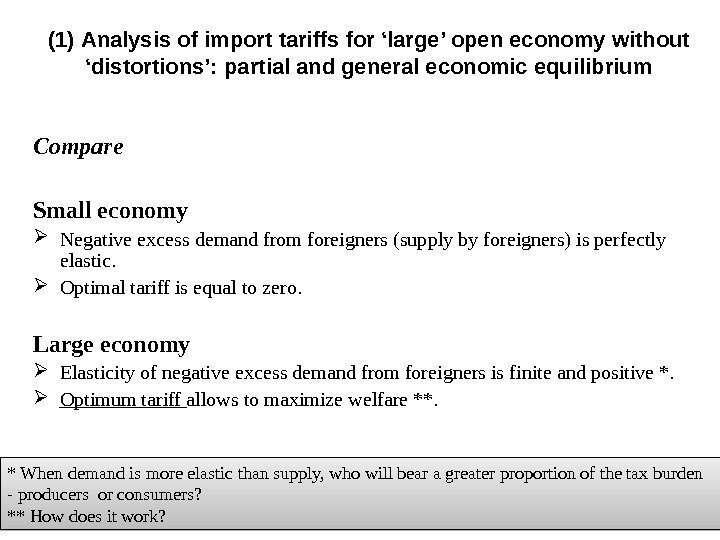( 1 ) Analysis of import tariffs for ‘large’ open economy without ‘distortions’: partial and general economic equilibrium Compare Small economy Negative excess demand from foreigners (supply by foreigners) is perfectly elastic. Optimal tariff is equal to zero. Large economy Elasticity of negative excess demand from foreigners is finite and positive *. Optimum tariff allows to maximize welfare **. * When demand is more elastic than supply, who will bear a greater proportion of the tax burden — producers or consumers? ** How does it work?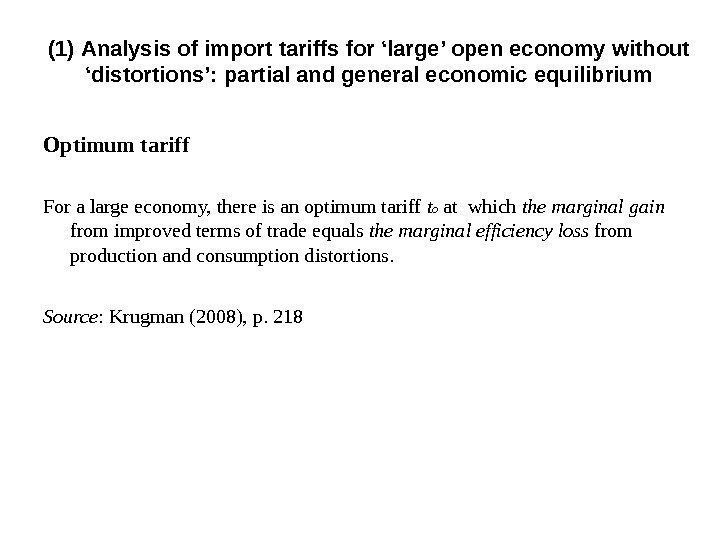Optimum tariff For a large economy, there is an optimum tariff t o at which the marginal gain from improved terms of trade equals the marginal efficiency loss from production and consumption distortions. Source : Krugman (2008), p. 218( 1 ) Analysis of import tariffs for ‘large’ open economy without ‘distortions’: partial and general economic equilibrium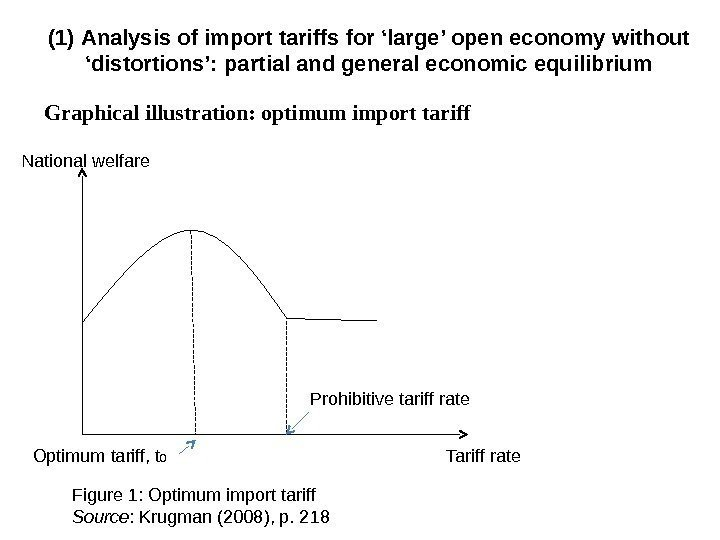Graphical illustration: optimum import tariff ( 1 ) Analysis of import tariffs for ‘large’ open economy without ‘distortions’: partial and general economic equilibrium Figure 1: Optimum import tariff Source : Krugman (2008), p. 218 National welfare Tariff rate. Prohibitive tariff rate Optimum tariff, t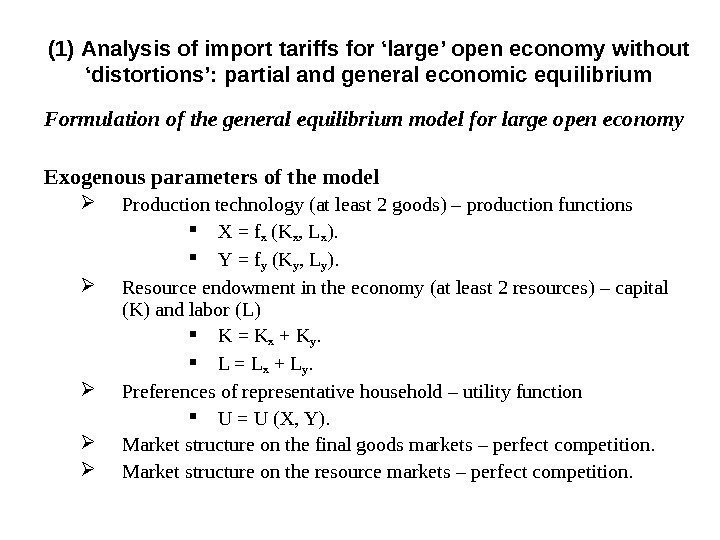Formulation of the general equilibrium model for large open economy Exogenous parameters of the model Production technology ( at least 2 goods ) – production functions Х = f x (K x , L x ). Y = f y (K y , L y ). Resource endowment in the economy ( at least 2 resources ) – capital ( K ) and labor ( L ) K = K x + K y. L = L x + L y. Preferences of representative household – utility function U = U (X, Y). Market structure on the final goods markets – perfect competition. Market structure on the resource markets – perfect competition. ( 1 ) Analysis of import tariffs for ‘large’ open economy without ‘distortions’: partial and general economic equilibrium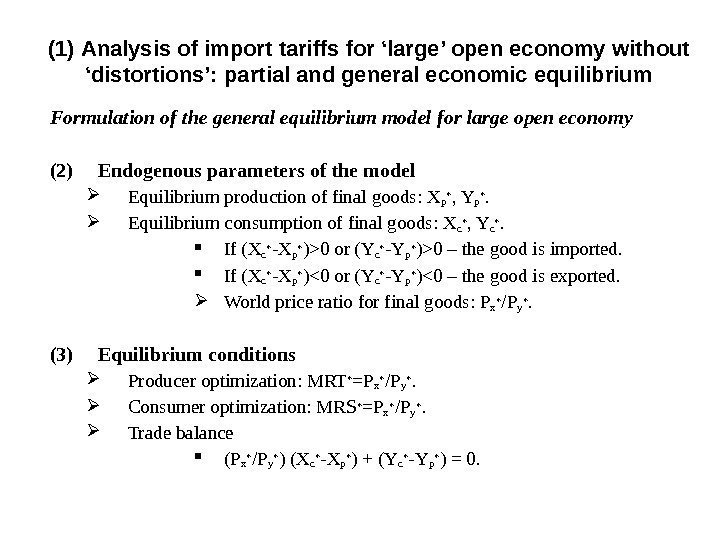Formulation of the general equilibrium model for large open economy (2) Endogenous parameters of the model Equilibrium production of final goods: X p * , Y p *. Equilibrium consumption of final goods : X c * , Y c *. If ( X c * — X p * ) > 0 or ( Y c * — Y p * ) > 0 – the good is imported. If ( X c * — X p * ) < 0 or ( Y c * — Y p * ) < 0 – the good is exported. World price ratio for final goods: P x * / P y *. (3) Equilibrium conditions Producer optimization : MRT * =P x * / P y *. Consumer optimization : MRS * =P x * / P y *. Trade balance ( P x * / P y * ) ( X c * — X p * ) + ( Y c * — Y p * ) = 0. ( 1 ) Analysis of import tariffs for ‘large’ open economy without ‘distortions’: partial and general economic equilibrium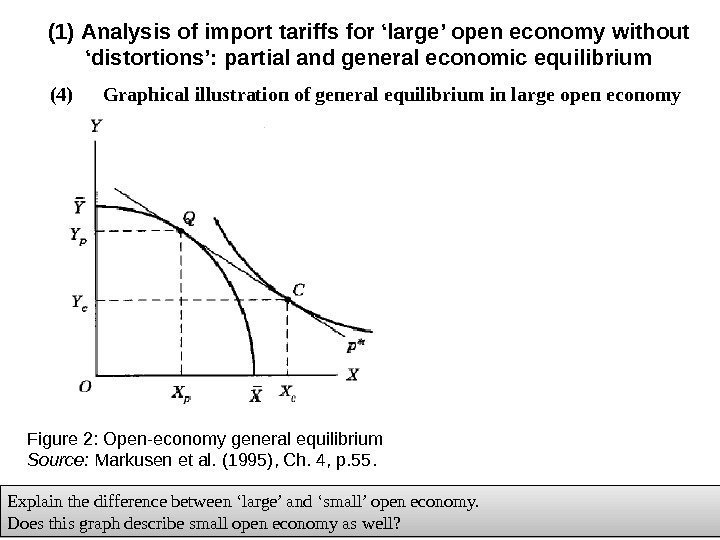(4) Graphical illustration of general equilibrium in large open economy( 1 ) Analysis of import tariffs for ‘large’ open economy without ‘distortions’: partial and general economic equilibrium Explain the difference between ‘large’ and ‘small’ open economy. Does this graph describe small open economy as well? Figure 2: Open-economy general equilibrium Source: Markusen et al. (1995), Ch. 4, p. 55.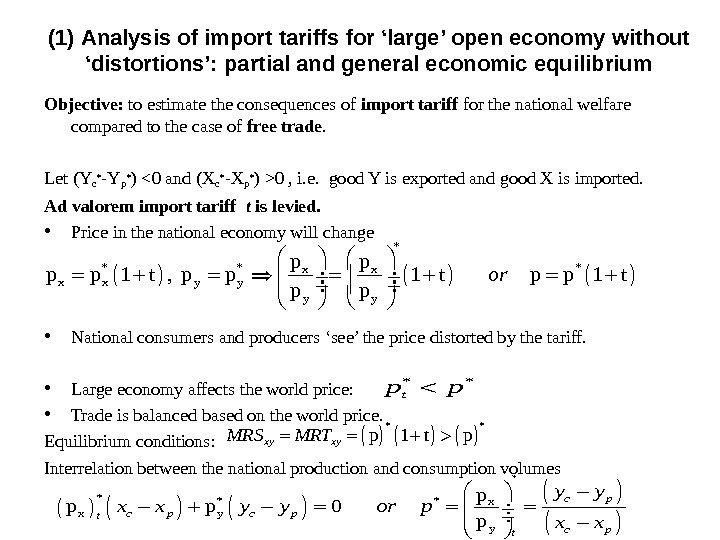Objective: to estimate the consequences of import tariff for the national welfare compared to the case of free trade. Let ( Yc* — Yp* ) 0 , i. e. good Y is exported and good X is imported. Ad valorem import tariff t is levied. • Price in the national economy will change • National consumers and producers ‘see’ the price distorted by the tariff. • Large economy affects the world price: • Trade is balanced based on the world price. Equilibrium conditions : Interrelation between the national production and consumption volumes * *x x y y p p 1 t , p p 1 t p p or * * p 1 t p xy xy. MRS MRT * ** *x x y y p p p 0 p c p c pt y y x x y y or p x x ( 1 ) Analysis of import tariffs for ‘large’ open economy without ‘distortions’: partial and general economic equilibrium * * tp p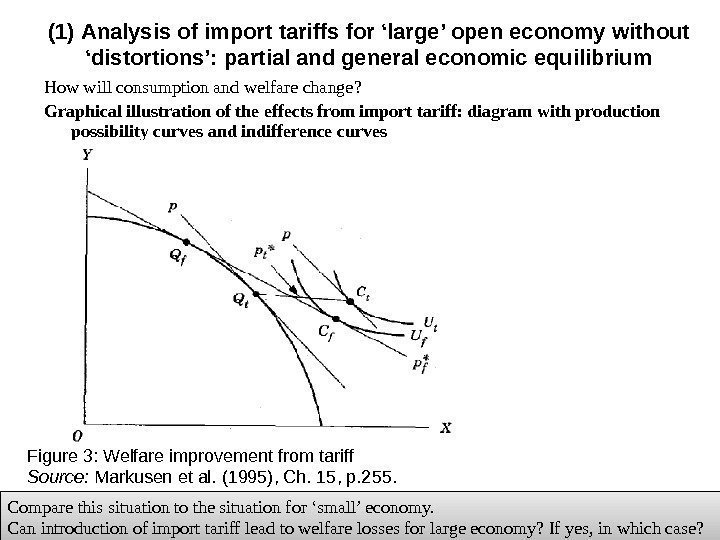How will consumption and welfare change ? Graphical illustration of the effects from import tariff: diagram with production possibility curves and indifference curves( 1 ) Analysis of import tariffs for ‘large’ open economy without ‘distortions’: partial and general economic equilibrium Figure 3: Welfare improvement from tariff Source: Markusen et al. (1995), Ch. 15, p. 255. Compare this situation to the situation for ‘small’ economy. Can introduction of import tariff lead to welfare losses for large economy? If yes, in which case?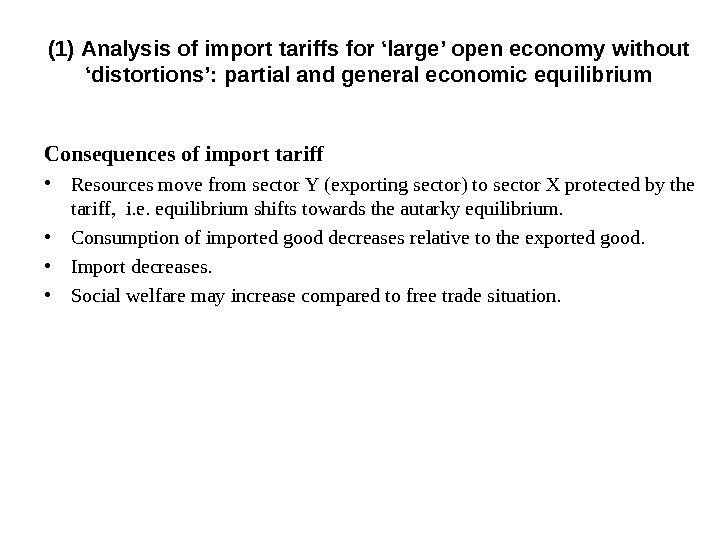Consequences of import tariff • Resources move from sector Y ( exporting sector ) to sector X protected by the tariff , i. e. equilibrium shifts towards the autarky equilibrium. • Consumption of imported good decreases relative to the exported good. • Import decreases. • Social welfare may increase compared to free trade situation. ( 1 ) Analysis of import tariffs for ‘large’ open economy without ‘distortions’: partial and general economic equilibrium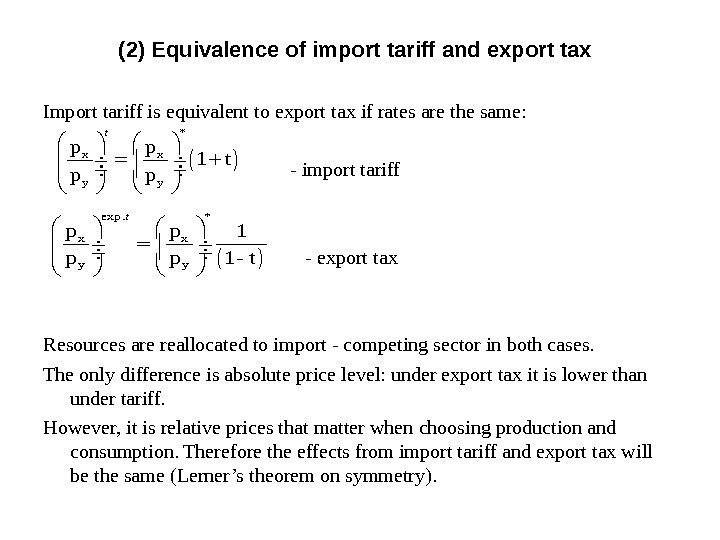(2) Equivalence of import tariff and export tax Import tariff is equivalent to export tax if rates are the same: — import tariff — export tax Resources are reallocated to import — competing sector in both cases. The only difference is absolute price level: under export tax it is lower than under tariff. However, it is relative prices that matter when choosing production and consumption. Therefore the effects from import tariff and export tax will be the same (Lerner’s theorem on symmetry). * x x y y p p 1 t p p t exp. * x x y y p p 1 — t t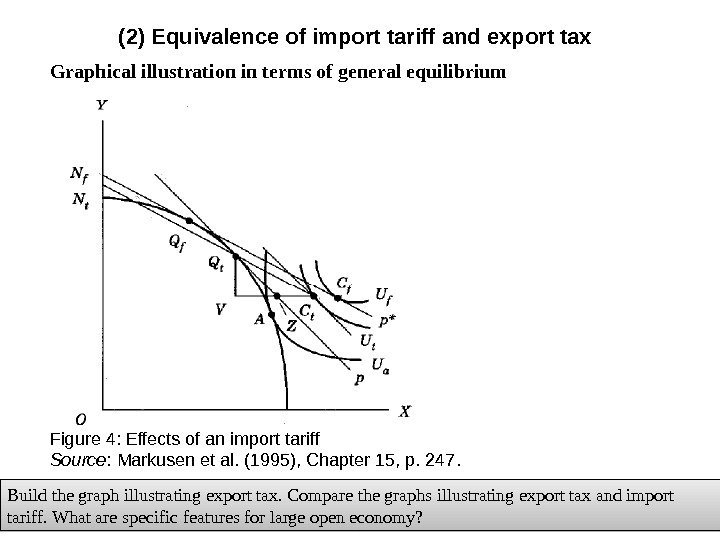(2) Equivalence of import tariff and export tax Graphical illustration in terms of general equilibrium Build the graph illustrating export tax. Compare the graphs illustrating export tax and import tariff. What are specific features for large open economy? Figure 4: Effects of an import tariff Source : Markusen et al. (1995) , Chapter 15, p. 247.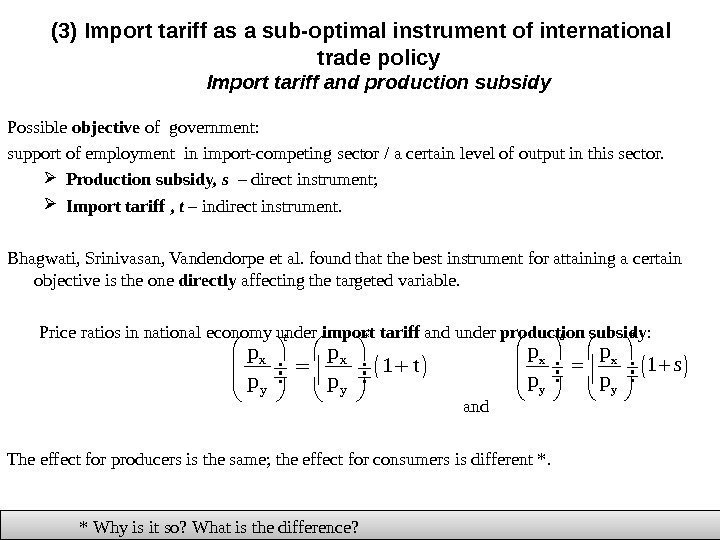(3) Import tariff as a sub-optimal instrument of international trade policy Import tariff and production subsidy Possible objective of government: support of employment in import-competing sector / a certain level of output in this sector. Production subsidy, s – direct instrument; Import tariff , t – indirect instrument. Bhagwati, Srinivasan, Vandendorpe et al. found that the best instrument for attaining a certain objective is the one directly affecting the targeted variable. Price ratios in national economy under import tariff and under production subsidy : and The effect for producers is the same; the effect for consumers is different *. * Why is it so? What is the difference? * x x y y p p 1 t p p t * x x y yp p 1 p p s s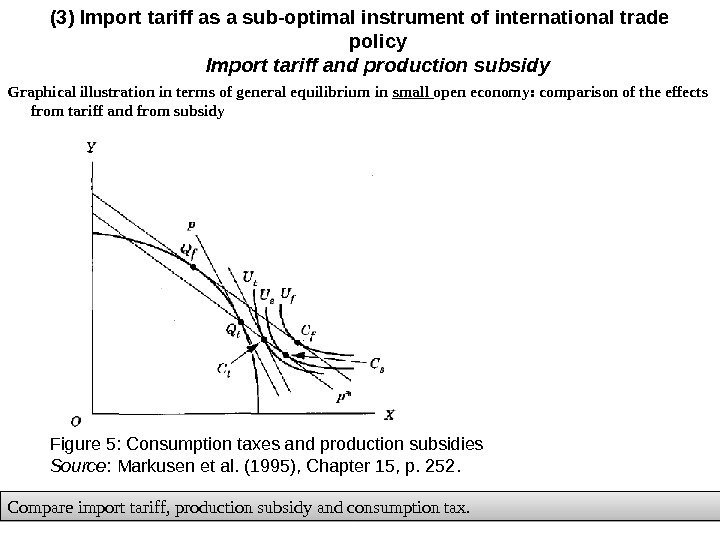Graphical illustration in terms of general equilibrium in small open economy: comparison of the effects from tariff and from subsidy (3) Import tariff as a sub-optimal instrument of international trade policy Import tariff and production subsidy Figure 5: Consumption taxes and production subsidies Source : Markusen et al. (1995) , Chapter 15, p. 252. Compare import tariff, production subsidy and consumption tax.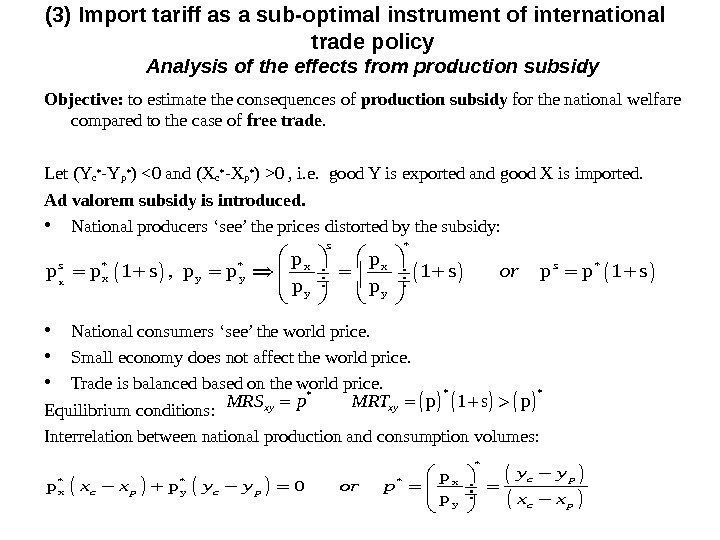Objective: to estimate the consequences of production subsidy for the national welfare compared to the case of free trade. Let ( Yc* — Yp* ) 0 , i. e. good Y is exported and good X is imported. Ad valorem subsidy is introduced. • National producers ‘see’ the prices distorted by the subsidy: • National consumers ‘see’ the world price. • Small economy does not affect the world price. • Trade is balanced based on the world price. Equilibrium conditions : Interrelation between national production and consumption volumes : x * *x x x y y p p 1 s , p p 1 s p p s s sor * **p 1 s pxy xy. MRS p MRT * *xx y y pp p 0 p c p c p y y x x y y or p x x (3) Import tariff as a sub-optimal instrument of international trade policy Analysis of the effects from production subsidy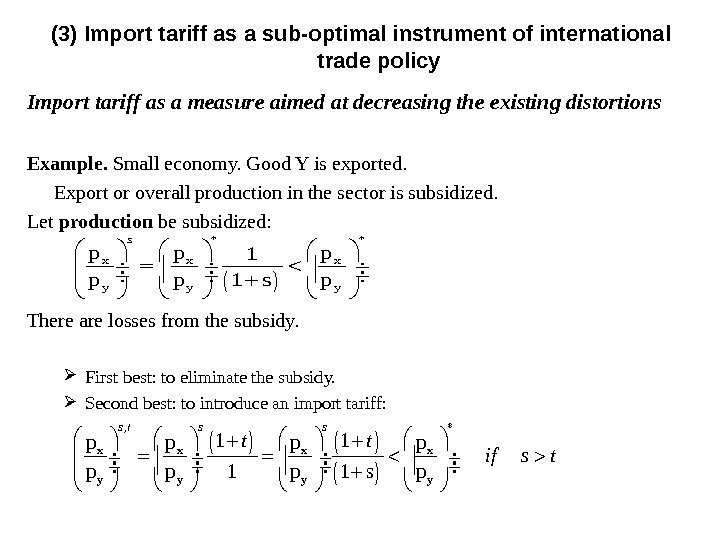Import tariff as a measure aimed at decreasing the existing distortions Example. Small economy. Good Y is exported. Export or overall production in the sector is subsidized. Let production be subsidized: There are losses from the subsidy. First best: to eliminate the subsidy. Second best: to introduce an import tariff: (3) Import tariff as a sub-optimal instrument of international trade policy * * x x x y y y p p p 1 s p s , * x x y y 1 1 p p p 1 p 1 s p s t s s t t if s t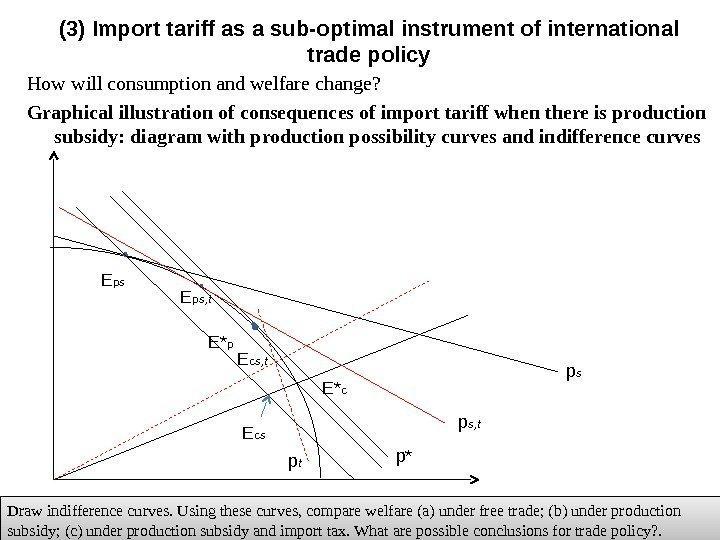How will consumption and welfare change ? Graphical illustration of consequences of import tariff when there is production subsidy: diagram with production possibility curves and indifference curves (3) Import tariff as a sub-optimal instrument of international trade policy p* p s. E* p. E p s E c s Draw indifference curves. Using these curves, compare welfare (a) under free trade; (b) under production subsidy; (c) under production subsidy and import tax. What are possible conclusions for trade policy? . p s, t. E p s, t p t. E c s, t E* c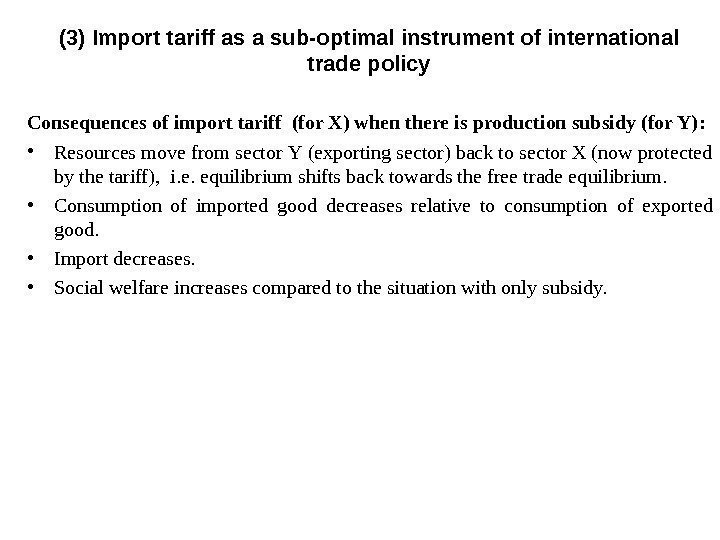Consequences of import tariff (for X) when there is production subsidy (for Y) : • Resources move from sector Y ( exporting sector ) back to sector X (now protected by the tariff) , i. e. equilibrium shifts back towards the free trade equilibrium. • Consumption of imported good decreases relative to consumption of exported good. • Import decreases. • Social welfare increases compared to the situation with only subsidy. (3) Import tariff as a sub-optimal instrument of international trade policy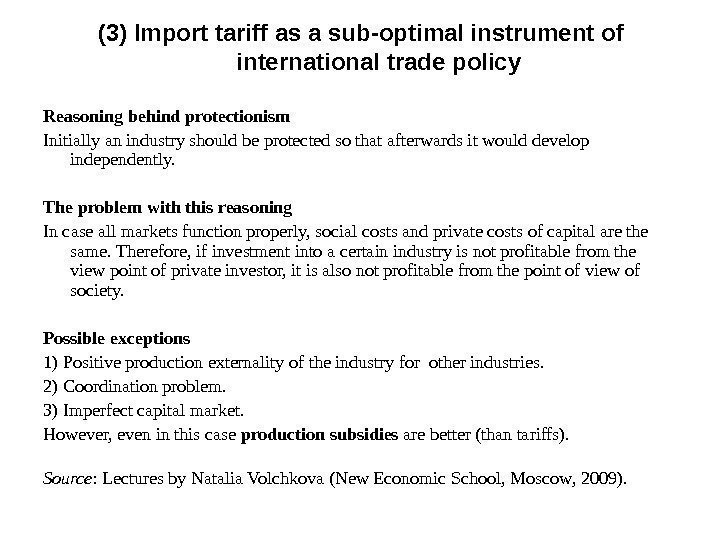Reasoning behind protectionism Initially an industry should be protected so that afterwards it would develop independently. The problem with this reasoning In case all markets function properly, social costs and private costs of capital are the same. Therefore, if investment into a certain industry is not profitable from the view point of private investor, it is also not profitable from the point of view of society. Possible exceptions 1) Positive production externality of the industry for other industries. 2) Coordination problem. 3) Imperfect capital market. However, even in this case production subsidies are better (than tariffs). Source : Lectures by Natalia Volchkova ( New Economic School, Moscow , 2 009). (3) Import tariff as a sub-optimal instrument of international trade policy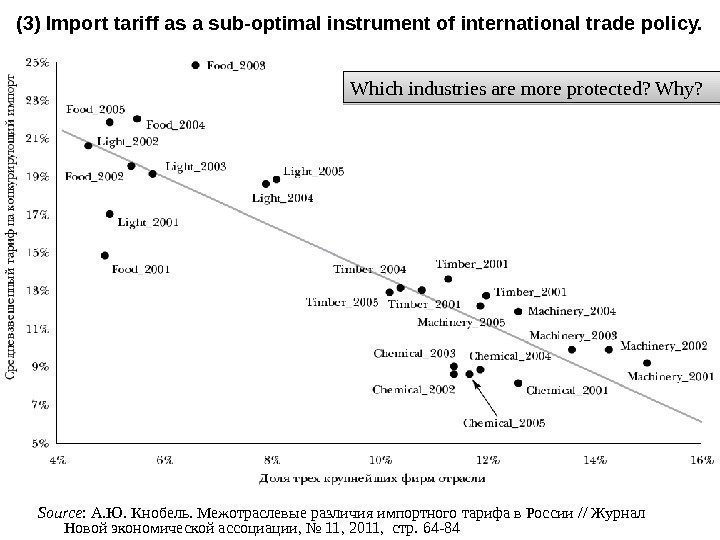Source : А. Ю. Кнобель. Межотраслевые различия импортного тарифа в России // Журнал Новой экономической ассоциации, № 11, 2011, стр. 64 -84 Which industries are more protected? Why? (3) Import tariff as a sub-optimal instrument of international trade policy.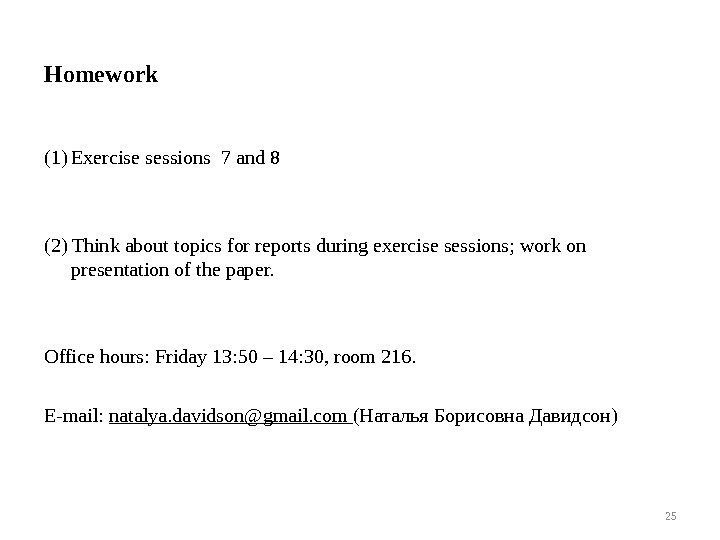(1) Exercise sessions 7 and 8 (2) Think about topics for reports during exercise sessions; work on presentation of the paper. Office hours: Friday 13: 50 – 14: 30, room 216. E-mail: natalya. [email protected] com (Наталья Борисовна Давидсон) 25 Homework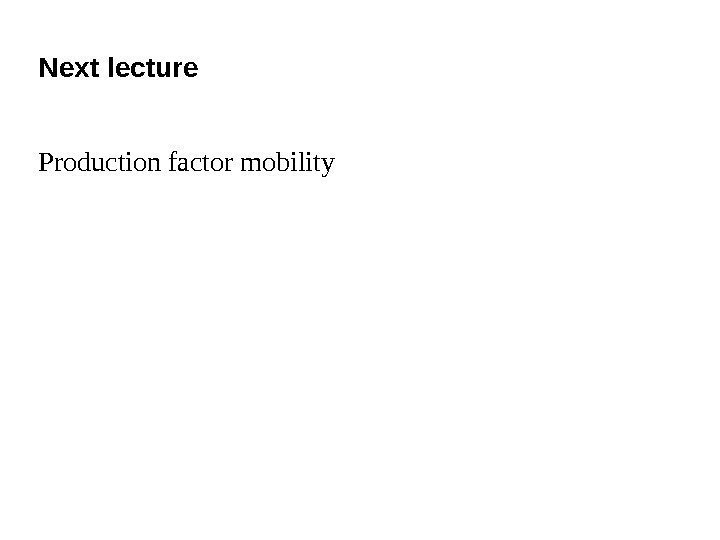Next lecture Production factor mobility